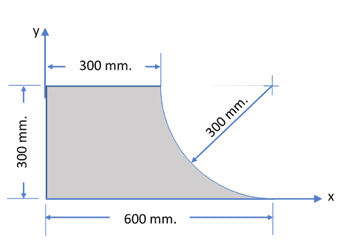# Mass moment of inertia of a composite shape

Homework Statement
What is the mass moment of inertia (kg-m^2) of the following steel plate about the x-axis
Relevant Equations
Mass moment of inertia of a quarter circular plate about its base is (1/4)mr^2
Mass moment of inertia of a rectangle along its base about the x-axis is(1/3)mh^2
thickness of the steel plate is 5mm
density of steel is 7850 kg/m^3My thought process was to get the mass moment of inertia of the rectangle and then subtract the mass moment of inertia of the quartercircle from it.
The MMoI of the rectangle is:
(1/3)(0.005*7850*.6*.3)(.3^2)= 0.212 meters
The MMoI of the quartercircle is:
(1/4)(0.005*7850*¼π 0.3^2)(.3^2) + ?
My problem is that I'm not sure how to apply the parallel axis theorem to get the Mass moment of inertia of the quarter circle about the x-axis.

Homework Helper
Gold Member
2022 Award
The MMoI of the rectangle is:
(1/3)(0.005*7850*.6*.3)(.3^2)= 0.212 meters

My problem is that I'm not sure how to apply the parallel axis theorem to get the Mass moment of inertia of the quarter circle about the x-axis.
Pay attention to units.

To use the parallel axis theorem you need to know where the mass centre is.

•I understand now, since I only know the mass moment of inertia about the base of the quartercircle, I first have to use this to find its mass moment of inertia about its centroid. Then I use this mass moment about the centroid to find the mass moment of inertia about the x-axis.
I have to apply the parallel axis theorem twice.

Homework Helper
Gold Member
2022 Award
I understand now, since I only know the mass moment of inertia about the base of the quartercircle, I first have to use this to find its mass moment of inertia about its centroid. Then I use this mass moment about the centroid to find the mass moment of inertia about the x-axis.
I have to apply the parallel axis theorem twice.
Yes.
I looked for a way to avoid this in the present case by using some symmetry arguments, but failed.

Homework Helper
Gold Member